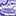# QlikView App Dev

Discussion Board for collaboration related to QlikView App Development.

Announcements
Customer & Partners, DEC. 9, 11 AM ET: Qlik Product & Strategy Roadmap Session: Data Analytics REGISTER NOW
cancel
Showing results for
Did you mean:Creator

## Nested if condition simplification

Hello experts,

Please have a look on the below part.

Table1:

if( len(Month)>2 and [Object Type Code]='A',(

if( ((LEFT((num#(Month)),4)='2010') AND (ApplyMap('PLSR_HISTORIC',VEHICLE_STATIC_ID)='REIT')),'CAL1' ,
IF( (LEFT((num#(Month)),4)<='2009'), 'CAL1'))),

if(len(Quarter)>2 and [Object Type Code]='A',(

if( ((NUM#(LEFT(Quarter,4))='2010') AND (ApplyMap('PLSR_HISTORIC',VEHICLE_STATIC_ID)='REIT')),'CAL1',
if( (NUM#(LEFT(Quarter,4))<='2009'),'CAL1'))))) as PLSR_CAL_HISTORIC,

if([Object Type Code] ='A' AND ((LEFT((num#(Month)),4)<='2010') OR (NUM#(LEFT(Quarter,4))<='2010')) and
// (ASSET_STATIC_ID='10649' or ASSET_STATIC_ID='10650' or ASSET_STATIC_ID='10651' or ASSET_STATIC_ID='10660'),'RTN',
wildmatch(ASSET_STATIC_ID,'10649','10650','10651','10660'),'RTN',

if( len(Month)>2 and [Object Type Code]='A',(

if( ((LEFT((num#(Month)),4)='2010') AND (ApplyMap('PLSR_HISTORIC',VEHICLE_STATIC_ID)='REIT')),'CAL1',
IF( (LEFT((num#(Month)),4)<='2009'), 'CAL1'))),

if(len(Quarter)>2 and [Object Type Code]='A',(

if( ((NUM#(LEFT(Quarter,4))='2010') AND (ApplyMap('PLSR_HISTORIC',VEHICLE_STATIC_ID)='REIT')),'CAL1',
if( (NUM#(LEFT(Quarter,4))<='2009'),'CAL1')))))) as ILSR_CAL_HISTORIC,

if([Object Type Code] ='A' AND ((LEFT((num#(Month)),4)<='2010') OR (NUM#(LEFT(Quarter,4))<='2010')) and

wildmatch(ASSET_STATIC_ID,'10649','10650','10651','10660'),'TOTAL FOR RTN',

//(ASSET_STATIC_ID='10649' or ASSET_STATIC_ID='10650' or ASSET_STATIC_ID='10651' or ASSET_STATIC_ID='10660'),'TOTAL FOR RTN',

if( len(Month)>2 and [Object Type Code]='A',(

if( ((LEFT((num#(Month)),4)='2010') AND (ApplyMap('PLSR_HISTORIC',VEHICLE_STATIC_ID)='REIT')),'TOTAL FOR NON-RTN',
IF( (LEFT((num#(Month)),4)<='2009'), 'TOTAL FOR NON-RTN'))),

if(len(Quarter)>2 and [Object Type Code]='A',(

if( ((NUM#(LEFT(Quarter,4))='2010') AND (ApplyMap('PLSR_HISTORIC',VEHICLE_STATIC_ID)='REIT')),'TOTAL FOR NON-RTN',
if( (NUM#(LEFT(Quarter,4))<='2009'),'TOTAL FOR NON-RTN')))))) as ILSR_CAL_HISTORIC2

from t1;

Can you please suggest how to simplify  and reduce the above mentioned code.

Thanks

Labels (4)

• ### Scripting

0 RepliesCommunity Browser